# Moment of inertia about tangent of a hoop

The hoop has radius R.

I used the same way to plot the axis for the hoop: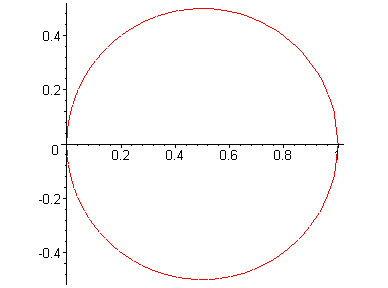##l^2 = r^2= 4R^2cos\theta##
since: ##r=2Rcos\theta##

$$2\rho \int_{0}^{\frac{\pi}{2}} r^2 r d\theta$$
$$2\rho \int_{0}^{\frac{\pi}{2}} 8R^3 cos^3\theta d\theta$$

answer is $$\frac{32}{3}R^3\rho$$ , and its wrong

using the same method to find mass is also wrong, which suggest a fundamental mistake in my solution but I don't know what it is.
$$2\rho \int_{0}^{\frac{\pi}{2}} r d\theta$$
$$2\rho \int_{0}^{\frac{\pi}{2}} 2R cos\theta d\theta$$
that's equal $$4\pi r$$ which is wrong

what is wrong with my solution?, it was really bizarre when I found that the mass itself using the limits I found actually gives a wrong answer.

What did I miss?

Last edited:

## Answers and Replies

Don't know why but latex is not working for nearly every equation. I did check equations validity while writing the post with no hope.

SteamKing
Staff Emeritus
Science Advisor
Homework Helper
Don't know why but latex is not working for nearly every equation. I did check equations validity while writing the post with no hope.

Take out the inline Latex (##) and convert to regular LaTex.

Please post the full text of the original Problem Statement.

SammyS
Staff Emeritus
Science Advisor
Homework Helper
Gold Member
Don't know why but latex is not working for nearly every equation. I did check equations validity while writing the post with no hope.
Get rid of the Bold tags.

By the way you should state in the body of the thread what is the problem which you are trying to solve. Do this no matter what you state in the thread title.

Get rid of the Bold tags.

By the way you should state in the body of the thread what is the problem which you are trying to solve. Do this no matter what you state in the thread title.

Take out the inline Latex (##) and convert to regular LaTex.

Please post the full text of the original Problem Statement.

The question is general, they just want a general solution. Question: "What is the moment of inertia about a tangent of a circle of radius R, density ρ"

---------------------------------------------------------------------------------
The hoop has radius R.

I used the same way to plot the axis for the hoop "So that y-axis is the tangent":##l^2 = x^2= 4R^2cos^4\theta##
since: ##r=2Rcos\theta## & ##cos\theta = \frac{x}{r} = \frac{r}{2R}##

$$2\rho \int_{0}^{\frac{\pi}{2}} x^2 r d\theta$$
$$2\rho \int_{0}^{\frac{\pi}{2}} 8R^3 cos^5\theta d\theta$$

answer is $$\frac{128}{15}R^3\rho$$ , and its wrong

using the same method to find mass is also wrong, which suggest a fundamental mistake in my solution but I don't know what it is.
$$2\rho \int_{0}^{\frac{\pi}{2}} r d\theta$$
$$2\rho \int_{0}^{\frac{\pi}{2}} 2R cos\theta d\theta$$
that's equal $$4\pi r \rho$$ which is wrong

what is wrong with my solution?, it was really bizarre when I found that the mass itself using the limits I found actually gives a wrong answer.

What did I miss?

Last edited:
Do you know the parallel axis , and perpendicular axis theorem ?

Nidum
Science Advisor
Gold Member
Hoop has no volume and no mass so question as given is meaningless ?

Hoop has no volume and no mass so question as given is meaningless ?
A 2d figure can't have mass ?

STEMucator
Homework Helper
Judging from the title and the information you have provided, you want to find the moment of inertia about the ##y##-axis of a circular lamina. The moment of inertia about the ##y##-axis is given by:

$$\iint_D x^2 \rho(x, y) \space dA$$

Using polar co-ordinates, the limits for the integral should look like ##0 \leq \theta \leq 2 \pi## and ##0 \leq r \leq R##.

You are also told the circular lamina has density ##\rho##. I assume this means the density is constant and can be pulled outside of the integral.

You can find the mass afterwards using:

$$\text{mass = density x area}$$

SteamKing
Staff Emeritus
Science Advisor
Homework Helper
Judging from the title and the information you have provided, you want to find the moment of inertia about the ##y##-axis of a circular lamina...

Except, the word "hoop" implies something with a hole in the middle, like a skinny torus:

http://yorkshirefitnesshire.co.uk/wp-content/uploads/2012/11/hula-hoop.jpg [Broken]​

Last edited by a moderator:
STEMucator
Homework Helper
Except, the word "hoop" implies something with a hole in the middle, like a skinny torus:

http://yorkshirefitnesshire.co.uk/wp-content/uploads/2012/11/hula-hoop.jpg [Broken]​

True, but from the picture the OP has given in the previous posts, it appears as if we are looking at the projection of the hoop on the ##xy##-plane. I guess "lamina" wouldn't be the right term. Replacing all instances of the word "lamina" with "hoop" in my prior post should fix things.

The OP posted the question:

The question is general, they just want a general solution. Question: "What is the moment of inertia about a tangent of a circle of radius R, density ρ"

I was specifically trying to find some relation to the statement "What is the moment of inertia about a tangent of a circle of radius R, density ρ".

Perhaps this is not even the actual problem statement. I would personally find it hard to believe the problem statement was given as is.

Last edited by a moderator:
Except, the word "hoop" implies something with a hole in the middle, like a skinny torus:

http://yorkshirefitnesshire.co.uk/wp-content/uploads/2012/11/hula-hoop.jpg [Broken]​

True, but from the picture the OP has given in the previous posts, it appears as if we are looking at the projection of the hoop on the ##xy##-plane. I guess "lamina" wouldn't be the right term. Replacing all instances of the word "lamina" with "hoop" in my prior post should fix things.

The OP posted the question:

I was specifically trying to find some relation to the statement "What is the moment of inertia about a tangent of a circle of radius R, density ρ".

Perhaps this is not even the actual problem statement. I would personally find it hard to believe the problem statement was given as is.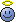Seems like I made a confusing question for you all ><.

We have a hoop of radius R, the question is to find the moment of inertia on a tangent to the hoop "any tangent will have the same moment of inertia".

The plot was just to show that I didn't draw the center of the "hoop" at the origin, instead I draw it as the plot show "center (R,0)", so that I can find the moment of inertia about y-axis, since now y-axis is the tangent of the hoop.

part (b):

http://im88.gulfup.com/2BPadu.png [Broken]

Last edited by a moderator:
SteamKing
Staff Emeritus
Science Advisor
Homework HelperSeems like I made a confusing question for you all ><.

We have a hoop of radius R, the question is to find the moment of inertia on a tangent to the hoop "any tangent will have the same moment of inertia".

The plot was just to show that I didn't draw the center of the "hoop" at the origin, instead I draw it as the plot show "center (R,0)", so that I can find the moment of inertia about y-axis, since now y-axis is the tangent of the hoop.

part (b):

http://im88.gulfup.com/2BPadu.png [Broken]

This is why the Rules of PF Homework Forums ask people to post the text of the entire question.

The word "hoop" accurately describes the object for which the moment of inertia is to be calculated, but the plot the OP made showing a circle which is tangent to the y-axis is ambiguous.

Some people thought the question was asking the student to calculate the MOI of a thin 2-dimensional region, rather than a wire bent as described.

Last edited by a moderator:
•Nidum, STEMucator, SammyS and 1 other person
STEMucator
Homework Helper
Now that the question has been properly established, we can find the real answer. For anyone posting needing help, please be clear about your concerns. Communication really is more important than anything, especially if you want help.

If the object is a 3D torus rather than a 2D circle, the moment of inertia can be found using triple integration rather than double integration.

Lets do part b) since you were asking about it. Rather than doing the integration about a co-ordinate axis, the integration is done over a co-ordinate plane. Since the object is a torus, it wont matter too much what plane you choose.

Lets choose the x-plane since the x-plane is tangent to the torus (so are the two other planes). The moment of inertia will be given by:

$$M_{x} = \iiint_E x \rho(x, y, z) \space dV$$

The mass is given by:

$$m = \iiint_E \rho(x, y, z) \space dV$$

There is a much simpler formula than the integral above, which can be used to compute the mass more quickly.

Although I still disagree with the problem statement, it called it a 'tangent line' rather than a 'tangent plane'.

SammyS
Staff Emeritus
Science Advisor
Homework Helper
Gold Member
... Since the object is a torus, it won't matter too much what plane you choose.
...

Lets choose the x-plane since the x-plane is tangent to the torus (so are the two other planes). The moment of inertia will be given by:
...

There is a much simpler formula than the integral above, which can be used to compute the mass more quickly.

Although I still disagree with the problem statement, it called it a 'tangent line' rather than a 'tangent plane'.
It's clear that it's a wire bent into the shape of a circle. It's a wire so it would seem likely that we can ignore the thickness of the wire. No need consider it as a torus.

As per the figure given in the OP, the axis of rotation is the y-axis, the y-axis being tangent to the circular hoop.

How can you have a moment of inertia about a plane? What does that even mean ? Also, what do you mean by the " x-plane " ?

@ahmed markhoos ,

It may simplify things to find the moment of inertia about the line, x = 0.5, then use the parallel axis theorem .

Last edited:
•Nidum
STEMucator
Homework Helper
It's clear that it's a wire bent into the shape of a circle. It's a wire so it would seem likely that we can ignore the thickness of the wire. No need consider it as a torus.

As per the figure given in the OP, the axis of rotation is the y-axis, the y-axis being tangent to the circular hoop.

How can you have a moment of inertia about a plane? What does that even mean ? Also, what do you mean by the " x-plane " ?

@ahmed markhoos ,

It may simplify things to find the moment of inertia about the line, x = 0.5, then use the parallel axis theorem .

Yes, the wire is a solid object with density, which has been looped. Lets not be took picky about the imaginative process since thickness isn't relevant anyway. I like imagining a fat loop, perhaps you enjoy a skinny wire.

Wait what? We take moments of inertia about co-ordinate planes. Perhaps I was unclear, I should have written the moment as:

$$M_{yz} = \iiint_E x \rho(x, y, z) \space dV$$

and said the yz-plane, not the x-plane. I guess I was looking at the x in the integral. In any case, if we want to do a moment about the y-axis through the xy-plane, we would just use:

$$M_{xy} = \iiint_E z \rho(x, y, z) \space dV$$

We could have found the moment of inertia using any co-ordinate plane and gotten the same answer because of symmetry anyway.

EDIT: I found a textbook reference that should make what I'm saying more reasonable to you: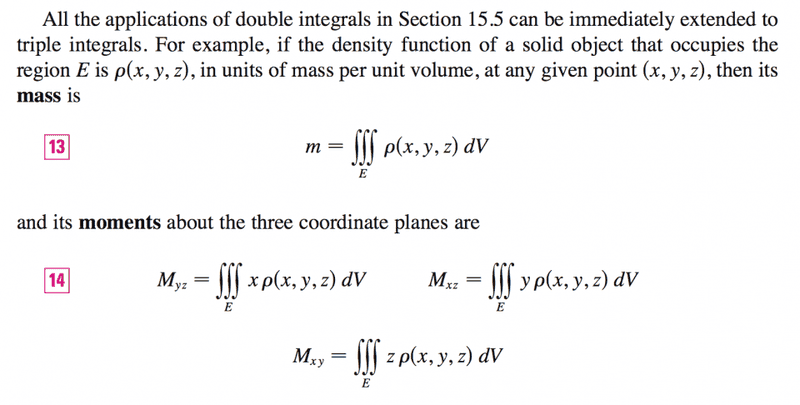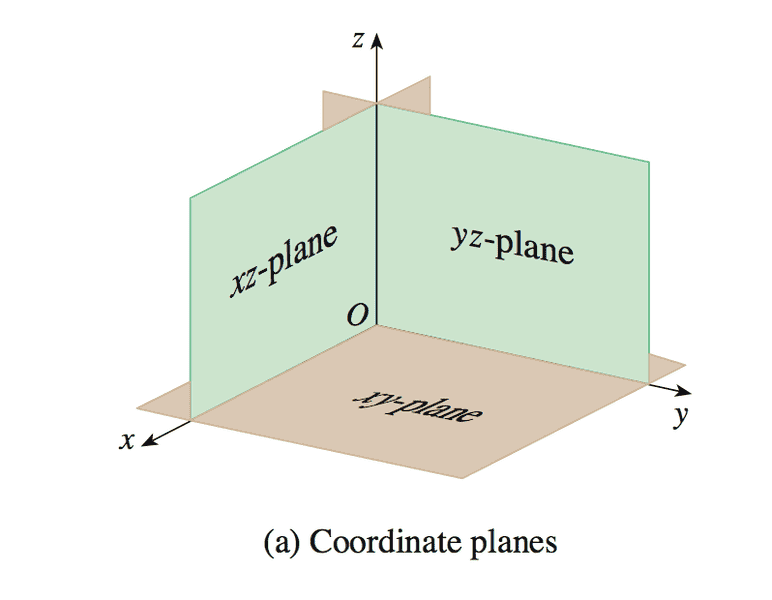Last edited:
SammyS
Staff Emeritus
Science Advisor
Homework Helper
Gold Member
Yes, the wire is a solid object with density, which has been looped. Lets not be took picky about the imaginative process since thickness isn't relevant anyway. I like imagining a fat loop, perhaps you enjoy a skinny wire.

Wait what? We take moments of inertia about co-ordinate planes. Perhaps I was unclear, I should have written the moment as:

$$M_{yz} = \iiint_E x \rho(x, y, z) \space dV$$

and said the yz-plane, not the x-plane. I guess I was looking at the x in the integral. In any case, if we want to do a moment about the y-axis through the xy-plane, we would just use:

$$M_{xy} = \iiint_E z \rho(x, y, z) \space dV$$

We could have found the moment of inertia using any co-ordinate plane and gotten the same answer because of symmetry anyway.

EDIT: I found a textbook reference that should make what I'm saying more reasonable to you:

View attachment 87700

View attachment 87701
Those are not moments of inertia. Those are first moments.a.k.a. moments of torque.

The moment of inertia is a second moment.

See this Wikipedia entry: https://en.wikipedia.org/wiki/Moment_(physics)#Elaboration

•Nidum
andrewkirk
Science Advisor
Homework Helper
Gold Member
The hoop has radius R.

I used the same way to plot the axis for the hoop "So that y-axis is the tangent":##l^2 = x^2= 4R^2cos^4\theta##
since: ##r=2Rcos\theta## & ##cos\theta = \frac{x}{r} = \frac{r}{2R}##

$$2\rho \int_{0}^{\frac{\pi}{2}} x^2 r d\theta$$
$$2\rho \int_{0}^{\frac{\pi}{2}} 8R^3 cos^5\theta d\theta$$

answer is $$\frac{128}{15}R^3\rho$$ , and its wrong

using the same method to find mass is also wrong, which suggest a fundamental mistake in my solution but I don't know what it is.
$$2\rho \int_{0}^{\frac{\pi}{2}} r d\theta$$
$$2\rho \int_{0}^{\frac{\pi}{2}} 2R cos\theta d\theta$$
that's equal $$4\pi r \rho$$ which is wrong

what is wrong with my solution?, it was really bizarre when I found that the mass itself using the limits I found actually gives a wrong answer.

What did I miss?
Why are you integrating from 0 to ##\frac{\pi}{2}## and what angle does your ##\theta## represent? If you are integrating over angles around the circle's centre your limits should be 0 to ##2\pi## and if you're integrating around angles at the origin your limits should be 0 to ##\pi## or ##-\frac{\pi}{2},\frac{\pi}{2}##.

In any case, for the tangent case I think it may be easier to use the x coordinate as your integration variable instead of an angle. Limits would be 0 to 2R.

andrewkirk
Science Advisor
Homework Helper
Gold Member
I take it back about using x as an integration variable. It's easy to do as an integral over ##\theta## measured around the centre of the circle, from 0 to ##2\pi##. The integrand will be a quadratic in ##\cos\theta##, which is straightforward to integrate. Both my integrand and my limits are nothing like yours. How did you get yours?

STEMucator
Homework Helper
Those are not moments of inertia. Those are first moments.a.k.a. moments of torque.

The moment of inertia is a second moment.

See this Wikipedia entry: https://en.wikipedia.org/wiki/Moment_(physics)#Elaboration

I have found the correct section of the textbook: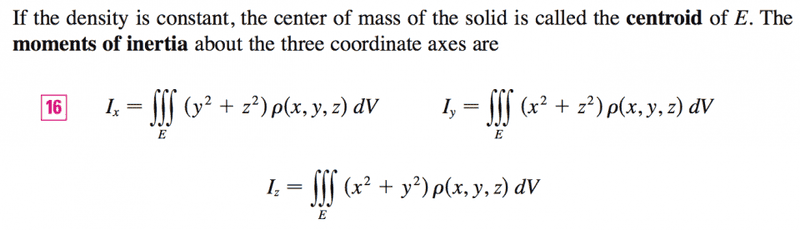I was extremely tired yesterday because I had zero hours of sleep. I probably shouldn't be trying to do math without being properly rested first.

Sorry about that.

•ahmed markhoos
Why are you integrating from 0 to ##\frac{\pi}{2}## and what angle does your ##\theta## represent? If you are integrating over angles around the circle's centre your limits should be 0 to ##2\pi## and if you're integrating around angles at the origin your limits should be 0 to ##\pi## or ##-\frac{\pi}{2},\frac{\pi}{2}##.

In any case, for the tangent case I think it may be easier to use the x coordinate as your integration variable instead of an angle. Limits would be 0 to 2R.

θ refers to the angle at the origin. Instead of writing ##-\frac{\pi}{2},\frac{\pi}{2}##. I just put it from 0 to ##\frac{\pi}{2}##. and multiplied the integration by 2.

andrewkirk
Science Advisor
Homework Helper
Gold Member
θ refers to the angle at the origin. Instead of writing ##-\frac{\pi}{2},\frac{\pi}{2}##. I just put it from 0 to ##\frac{\pi}{2}##. and multiplied the integration by 2.
That sounds good. Now, how did you get from $$2\rho \int_{0}^{\frac{\pi}{2}} x^2 r d\theta$$
to
$$2\rho \int_{0}^{\frac{\pi}{2}} 8R^3 cos^5\theta d\theta$$?
The first looks correct. The second does not.
If you show your working, someone can identify where you went wrong.

Hint: you have used ##r## in your first formula and ##R## in your second. Presumably the first is the radius of the circle. What is the second? Is it the same as ##r## and is it constant with respect to ##\theta##?

LCKurtz
Science Advisor
Homework Helper
Gold Member
That sounds good. Now, how did you get from $$2\rho \int_{0}^{\frac{\pi}{2}} x^2 r d\theta$$
to
$$2\rho \int_{0}^{\frac{\pi}{2}} 8R^3 cos^5\theta d\theta$$?
The first looks correct. The second does not.
If you show your working, someone can identify where you went wrong.

Hint: you have used ##r## in your first formula and ##R## in your second. Presumably the first is the radius of the circle. What is the second? Is it the same as ##r## and is it constant with respect to ##\theta##?

Lots of stuff wrong in this thread. The 2d formulas Zondrina is giving are irrelevant.

Also, the first equation in the quote above is not correct. Assuming ##\rho## is a linear density, the correct expression for the second moment would be$$\int x^2\rho~ds$$where everything is expressed in terms of ##\theta##. To set it up correctly you need the polar equation of the hoop in the form ##r = f(\theta)##, which I don't see where anyone has given, and from it the correct formula for ##ds##. It is not ##rd\theta##. Start by writing the polar equation of the hoop.

[Edit, added]: I see where the OP did write the polar equation of the hoop in post #5. His error there is not using the correct ##ds##.

Last edited:
andrewkirk
Science Advisor
Homework Helper
Gold Member
the first equation in the quote above is not correct
I agree. I hadn't noticed that, but while the formula would be right if ##\theta## were the angle at the centre of the circle and ##r## is the circle radius (because then ##ds=rd\theta##), it's not right when ##\theta## is the angle at the origin, which is what the OPer said.
It seems to me that integrating over angles at the centre of the circle is by far the easiest approach, because one gets a very simple integrand, and very little geometry is needed.

SteamKing
Staff Emeritus
Science Advisor
Homework Helper
Lots of stuff wrong in this thread. The 2d formulas Zondrina is giving are irrelevant.
In a thread this lengthy, it is not clear to which formulas you are referring.

The general formulas for moment of inertia given by Zondrina in Post #20 would be the correct ones to use.